#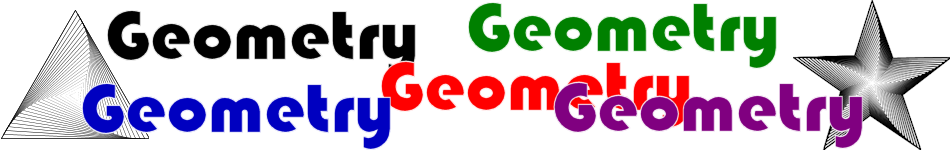There are 366 different Starters of The Day, many to choose from. You will find below some starters on the topic of Geometry. A lesson starter does not have to be on the same topic as the main part of the lesson or the topic of the previous lesson. It is often very useful to revise or explore other concepts by using a starter based on a totally different area of Mathematics.

Main Page

### Geometry Starters:Estimate the sizes of each of the angles then add your estimates together.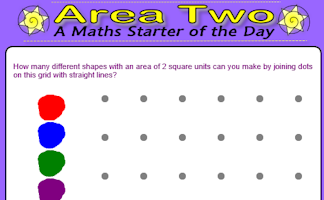How many different shapes with an area of 2 square units can you make by joining dots on this grid with straight lines?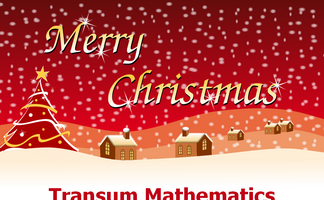Which of the two shapes has the largest area? You will be surprised!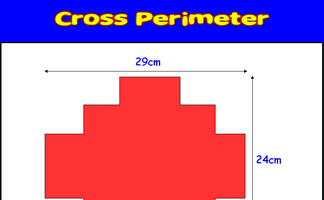Calculate the distance around the given shape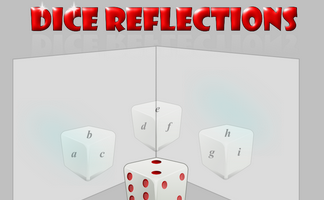A dice is reflected in two mirrors. What numbers can be seen?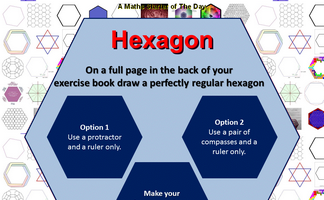On a full page in the back of your exercise book draw a perfectly regular hexagon.Memorise a picture made up of geometrical shapes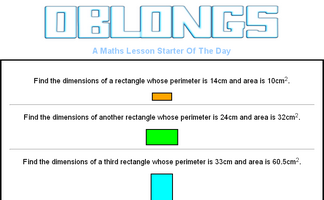Find the dimensions of a rectangle given the perimeter and area.On a full page in the back of your exercise book draw a perfectly regular pentagon.Solve the riddle to find the name of the polygon then sum the interior angles.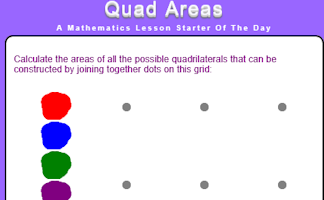Calculate the areas of all the possible quadrilaterals that can be constructed by joining together dots on this grid.On squared paper copy the drawing of the face then reflect it in three different lines.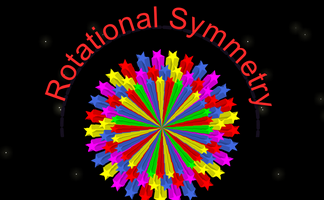Draw a pattern with rotational symmetry of order 6 but no line symmetry.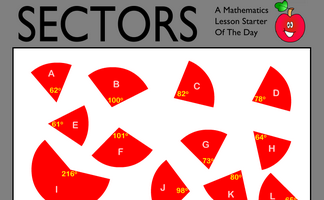Work out which sectors fit together to make complete circles. Knowledge of the sum of the angles at a point will help find more than one correct solution to this puzzle.Find a trapezium, a triangle and a quadrilateral where all of the angles are square numbers.Write down the names of all the mathematical shapes you know.Use the information implied in the diagram to calculate the perimeter of this shape.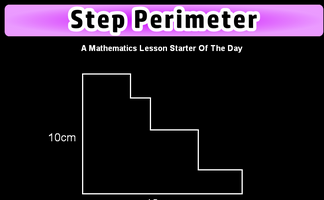Is it possible to work out the perimeter of this shape if not all the side lengths are given?

## Exercises#### Angle Chase

Find all of the angles on the geometrical diagrams.#### Angle Parallels

Understand and use the relationship between parallel lines and alternate and corresponding angles.#### Angle Points

Apply the properties of angles at a point, angles on a straight line and vertically opposite angles.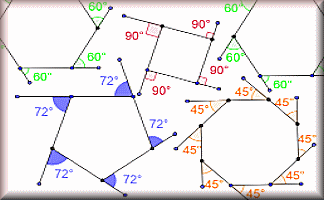#### Angle Theorems

Diagrams of the angle theorems with interactive examples.#### Angles in a Triangle

A self marking exercise involving calculating the unknown angle in a triangle.#### Area Maze

Use your knowledge of rectangle areas to calculate the missing measurement of these composite diagrams.#### Area of a Triangle

Calculate the areas of the given triangles in this self marking quiz.#### Area Two

How many different shapes with an area of 2 square units can you make by joining dots on this grid with straight lines?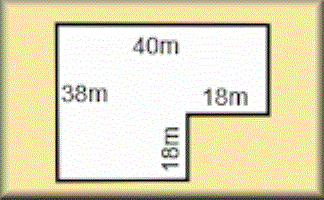#### Areas of Composite Shapes

Find the areas of combined (composite) shapes made up of one or more simple polygons and circles.#### Circle Parts Kim's Game

A memory game to be projected to help the whole class revise the names for the parts of a circle.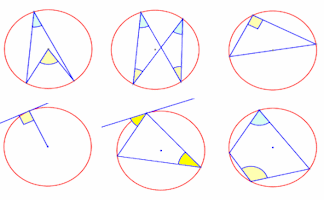#### Circle Theorems

Diagrams of the circle theorems to be projected onto a white board as an effective visual aid.#### Circle Theorems Exercise

Show that you understand and can apply the circle theorems with this self marking exercise.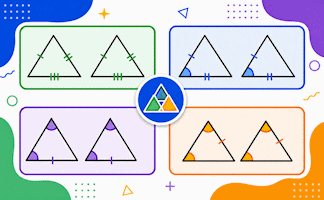#### Congruent Triangles

Test your understanding of the criteria for congruence of triangles with this self-marking quiz.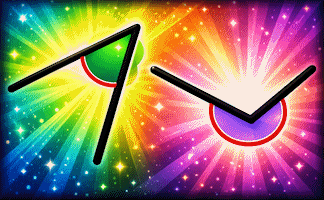#### Estimating Angles

Estimate the size of the given acute angles in degrees.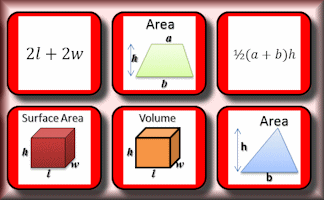#### Formulae Pairs

Find the matching pairs of diagrams and formulae for basic geometrical shapes.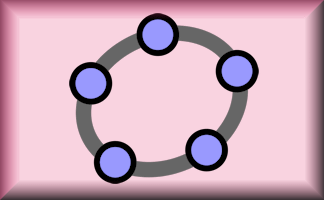#### Geometry Toolbox

Create your own dynamic geometrical diagrams using this truly amazing tool from GeoGebra.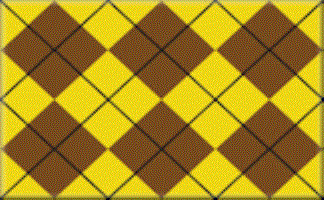#### How Many Rectangles?

Investigate the number of rectangles on a grid of squares. What strategies will be useful in coming up with the answer?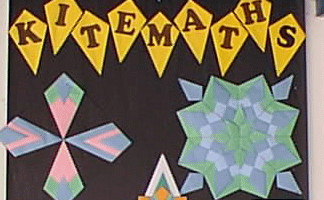#### Kite Maths

Can you make a kite shape from a single A4 size sheet of paper using only three folds?#### Maxvoltray

Find the maximum volume of a tray made from an A4 sheet of paper. A practical mathematical investigation.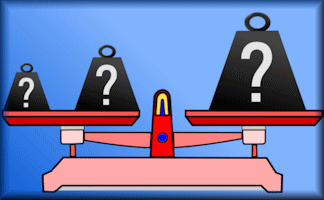#### Measuring Units

Check your knowledge of the units used for measuring with this multiple choice quiz about metric and imperial units.#### Pin Board

Rows and columns of dots that can be joined using straight lines to create shapes.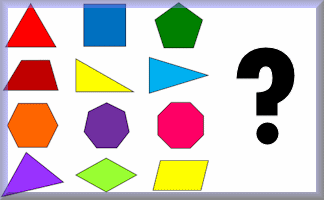#### Polybragging

The Transum version of the Top Trumps game played online with the properties of polygons.#### Polygon Angles

A mixture of problems related to calculating the interior and exterior angles of polygons.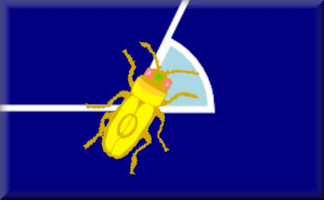#### Polygon Angles Animation

Barbara Bug walks around a regular hexagon turning through each of the exterior angles as she goes.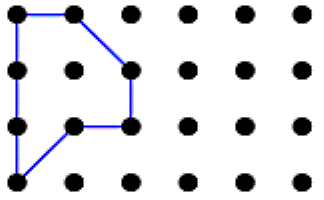#### Polygon Areas

Investigate polygons with an area of 4 sq. units. Investigate polygons with other areas.#### Polygon Pieces

Arrange the nine pieces of the puzzle on the grid to make different polygons.#### Polygon Properties

Connect the names of the polygons with the descriptions of their properties.#### Polygons

Name the polygons and show the number of lines and order of rotational symmetry.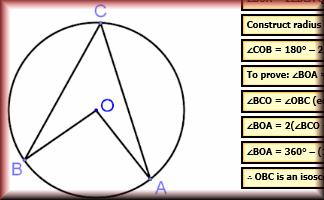#### Proof of Circle Theorems

Arrange the stages of the proofs for the standard circle theorems in the correct order.Calculate the areas of all the possible quadrilaterals that can be constructed by joining together dots on this grid.#### Rectangle Perimeters

The perimeter of a rectangle is 28cm. What could its area be?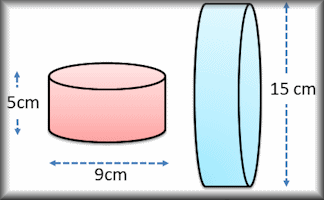#### Surface Area

Work out the surface areas of the given solid shapes.#### Transformations

Draw transformations online and have them instantly checked. Includes reflections, translations, rotations and enlargements.#### Vectors

An online exercise on addition and subtraction of vectors, multiplication of vectors by a scalar, and diagrammatic representations of vectors.#### Volume

Use formulae to solve problems involving the volumes of cuboids, cones, pyramids, prisms and composite solids.

### Search

The activity you are looking for may have been classified in a different way from the way you were expecting. You can search the whole of Transum Maths by using the box below.

Have today's Starter of the Day as your default homepage. Copy the URL below then select
Tools > Internet Options (Internet Explorer) then paste the URL into the homepage field.

Set as your homepage (if you are using Internet Explorer)

Do you have any comments? It is always useful to receive feedback and helps make this free resource even more useful for those learning Mathematics anywhere in the world. Click here to enter your comments.For All: